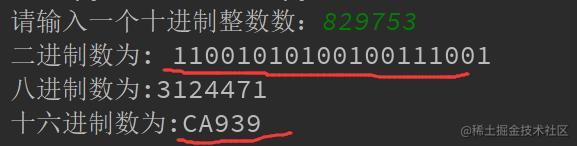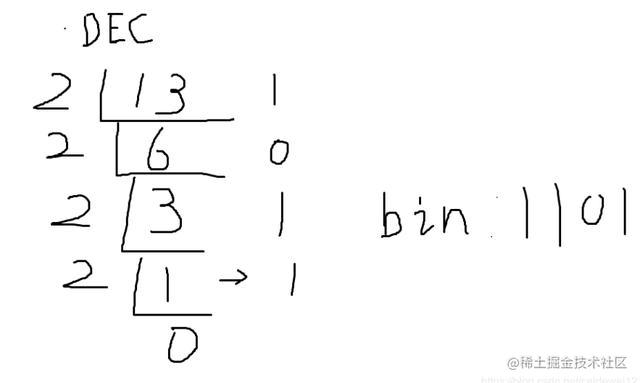# 一、导言

1. dec（十进制）—> bin（二进制）
2. dec（十进制）—> oct（八进制）
3. dec（十进制）—> hex（十六进制）

# 二、各种进制介绍``````#include<stdio.h>
#include<stdlib.h>
int main()
{
printf("rgb三原色转16进制\n");
int a,b,c,d;
while(d != 2){
printf("输入1继续，输入2退出\n");
scanf("%d",&d);
scanf("%d%d%d",&a,&b,&c);
printf("[%x %x %x]\n",a,b,c); //%x 可以直接把我们输入的十进制转换为 十六进制
}
return 0;
}

# 三、使用 Python 代码完成转换

3.1 十进制转换为二进制# 十进制整数转二进制

def dec_to_bin(num): #函数名做到见其名知其意

l = [] # 创建一个空列表

if num<0: # 是负数转换成整数

return "-"+ dec_to_bin(abs()) # 如过是负数，先转换成正数

while True:

num, reminder = divmod(num,2) # 短除法，对2求，分别得到除数 和 余数、这是 Python 的特有的一个内置方法，分别可以到商 及 余数

l.append(str(reminder)) # 把获得的余数 存入字符串

if num == 0: # 对应了前面的话，当商为 0时，就结束啦

return "".join(l[::-1]) # 对列表中的字符串进行逆序拼接，得到一个二进制字符串

3.2 十进制转八进制

``````# 十进制转八进制
def dec_to_oct(num):
l = []
if num<0:
return "-"+ dec_to_bin(abs(num)) # 如过是负数，先转换成正数
while True:
num, reminder = divmod(num,8) # 短除法，对2求，分别得到除数 和 余数、
l.append(str(reminder)) # 把获得的余数 存入字符串
if num == 0:
return "".join(l[::-1]) # 对列表中的字符串进行逆序拼接，得到一个二进制字符串

3.3 十进制转十六进制

``````# 十进制转十六进制（这个相对麻烦一点，因为，十六进制包含 A-F，大小写不敏感）
def dec_to_hex(num):
base = [str(x) for x in range(10)] +[chr(x) for x in range(ord('A'),ord("A")+6)] # 前者把 0 ~ 9 转换成字符串存进列表 base 里，后者把 A ~ F 存进列表
l = []
if num<0:
return "-"+dec_to_hex(abs(num))
while True:
num,rem = divmod(num,16) # 求商 和 留余数
l.append(base[rem])
if num == 0:
return "".join(l[::-1])